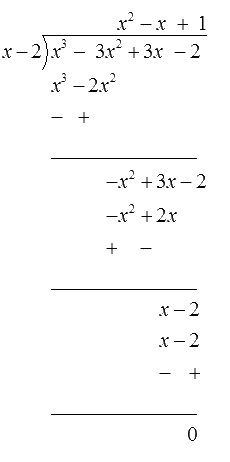# Ex.2.3 Q4 Polynomials Solution - NCERT Maths Class 10

Go back to  'Ex.2.3'

## Question

On dividing $$x^{3} -3 x^{2}+x +2$$ by a

polynomial $$g(x),$$ the quotient and remainder were $$x – 2$$ and $$–2x + 4,$$ respectively. Find $$g(x).$$

Video Solution
Polynomials
Ex 2.3 | Question 4

## Text Solution

What is unknown?

Divisor $$g(x)$$ of a polynomial $$p(x).$$

Reasoning:

This question is straight forward, you can solve it by using division algorithm

Dividend $$=$$ Divisor $$\times$$ Quotient $$+$$ Remainder

Put the given values in the above equation and simplify it, get the value of $$g(x).$$

Steps:

Dividend $$=$$ Divisor $$\times$$ Quotient $$+$$ Remainder

\begin{align}&x^{3}- 3 x^{2}\!+\!x\!+\!2 = \begin{bmatrix} g(x) \!\times\! x\! \\ -\!2\!+\!(-2 x\!+\!4) \end{bmatrix} \\& \begin{bmatrix} \left(x^{3}\!-\!3 x^{2}\!+\!x\!+\!2\right) \\ - (\!\!-\!2 x\!+\!4) \end{bmatrix} =\!g(x)\! \times\! x\!-\!2\\& \begin{bmatrix} x^{3}\!-\!3 x^{2}\!+\!x\!+\!2 x \\ +\!2\!-\!4\end{bmatrix} =\!g(x)\! \times\! x\!-\!2\\&\left(x^{3}\!-\!3 x^{2}\!+\!3 x\!-\!2\right)\!=\!g(x) \times x\!-\!2 \end{align}Therefore, $$g\left( x \right) = {x^2} - x + 1$$

Learn from the best math teachers and top your exams

• Live one on one classroom and doubt clearing
• Practice worksheets in and after class for conceptual clarity
• Personalized curriculum to keep up with school